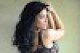# Calculate the GCD (greatest common divisor) and LCM (least common multiple) of two integers in C## GCD

The greatest common divisor of two integers is the largest integer that evenly divides them both. For example, GCD(180, 105) = 15 because 15 is the largest integer that divides evenly into both 180 and 105.

Euclid’s Elements c. 300 BC describes an efficient algorithm for calculating the greatest common divisor. The key idea is that the greatest common divisor of two numbers remains unchanged if you subtract the smaller from the larger.

The algorithm uses the fact that, if A >= B, then GCD(A, B) = GCD(B, A mod B). To calculate the greatest common divisor, you simply repeat this operation until A mod B is zero, at which point the greatest common divisor is B. Here’s the C# code:

```// Use Euclid's algorithm to calculate the
// greatest common divisor (GCD) of two numbers.
private long GCD(long a, long b)
{
a = Math.Abs(a);
b = Math.Abs(b);

// Pull out remainders.
for (; ; )
{
long remainder = a % b;
if (remainder == 0) return b;
a = b;
b = remainder;
};
}```

This algorithm is sometimes called the “Euclidean algorithm” or “Euclid’s algorithm.”

# LCM

The least common multiple (LCM) of two integers A and B is the smallest integer that is a multiple of both A and B. For example, LCM(36, 84) = 252 because 252 is the smallest integer into which both 36 and 84 divide evenly.

You can use the greatest common divisor to easily calculate the least common multiple. Suppose A = a * g and B = b * g where g = GCD(A, B) and a and b are the other factors of A and B. Then LCM(A, B) = A * B / LCM(A, B). Intuitively A * B contains two copies of the greatest common divisor (plus the other factors a and b) so you divide by the greatest common divisor to get the smallest multiple containing only 1 copy of the GCD.

Here’s the C# code:

```// Return the least common multiple
// (LCM) of two numbers.
private long LCM(long a, long b)
{
return a * b / GCD(a, b);
}```This entry was posted in algorithms, mathematics and tagged , , , , , , , , , , , , . Bookmark the permalink.

### 5 Responses to Calculate the GCD (greatest common divisor) and LCM (least common multiple) of two integers in C#

1.alex.a says:

recursive variant:

```private static int GCD(int a, int b)
{
if (a % b == 0)
{
return b;
}
return GCD(b, a % b);
}```
•RodStephens says:

You can make that even more concise:

```private static int GCD(int a, int b)
{
if (a % b == 0) return b;
return GCD(b, a % b);
}```
•ANON says:

private static int GCD(int a, int b)=>a%b==0?b:GCD(b,a%b)

Even more concise.